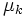# Difference between revisions of "Kinetic friction"

View a complete list of force types

## Definition

### Intensional definition

Kinetic friction, or dynamic friction, is defined as the form of friction between two bodies via their surface of contact (i.e., the force acting parallel to the plane of contact) when the two surfaces of contact are slipping against each other.

### Tendency-based definition

Kinetic friction is the form of friction that opposes the slipping against each other of two surfaces in contact. Its direction is opposite the direction of relative motion of the surfaces in contact. Note that it need not oppose the net external force between the surfaces in contact.

## Model

The typical model used for kinetic friction, which is applicable for small velocities, is the Coulomb model of friction, which states that the magnitude of kinetic friction is$\mu_kN$, where$\mu_k$ is the coefficient of kinetic friction (dependent on the nature of the surfaces in contact) and$N$ is the normal force between the bodies (measuring how hard they are pressed against each other).